Energy Basics

Learning Objectives

By the end of this section, you will be able to:

• Define energy, distinguish types of energy, and describe the nature of energy changes that accompany chemical and physical changes
• Distinguish the related properties of heat, thermal energy, and temperature
• Define and distinguish specific heat and heat capacity, and describe the physical implications of both
• Perform calculations involving heat, specific heat, and temperature change

Chemical changes and their accompanying changes in energy are important parts of our everyday world (Figure 1). The macronutrients in food (proteins, fats, and carbohydrates) undergo metabolic reactions that provide the energy to keep our bodies functioning. We burn a variety of fuels (gasoline, natural gas, coal) to produce energy for transportation, heating, and the generation of electricity. Industrial chemical reactions use enormous amounts of energy to produce raw materials (such as iron and aluminum). Energy is then used to manufacture those raw materials into useful products, such as cars, skyscrapers, and bridges.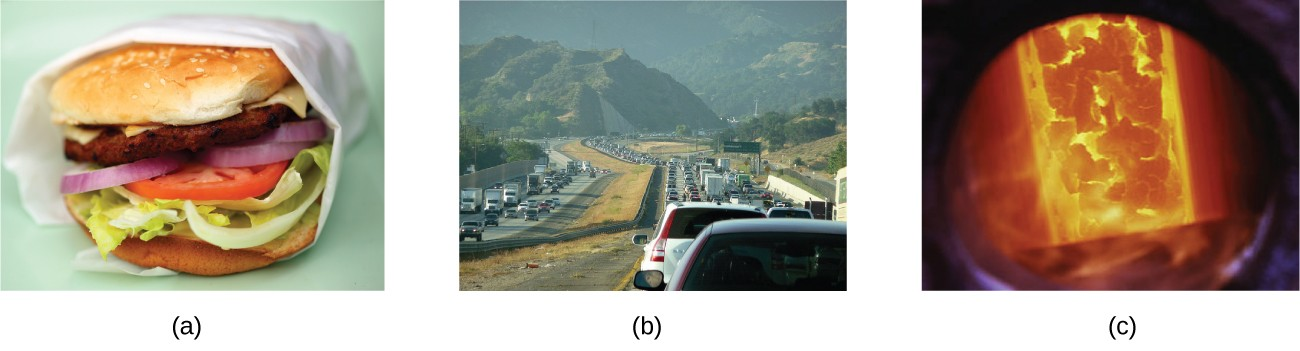Figure 1. The energy involved in chemical changes is important to our daily lives: (a) A cheeseburger for lunch provides the energy you need to get through the rest of the day; (b) the combustion of gasoline provides the energy that moves your car (and you) between home, work, and school; and (c) coke, a processed form of coal, provides the energy needed to convert iron ore into iron, which is essential for making many of the products we use daily. (credit a: modification of work by “Pink Sherbet Photography”/Flickr; credit b: modification of work by Jeffery Turner)

Over 90% of the energy we use comes originally from the sun. Every day, the sun provides the earth with almost 10,000 times the amount of energy necessary to meet all of the world’s energy needs for that day. Our challenge is to find ways to convert and store incoming solar energy so that it can be used in reactions or chemical processes that are both convenient and nonpolluting. Plants and many bacteria capture solar energy through photosynthesis. We release the energy stored in plants when we burn wood or plant products such as ethanol. We also use this energy to fuel our bodies by eating food that comes directly from plants or from animals that got their energy by eating plants. Burning coal and petroleum also releases stored solar energy: These fuels are fossilized plant and animal matter.

This chapter will introduce the basic ideas of an important area of science concerned with the amount of heat absorbed or released during chemical and physical changes—an area called thermochemistry. The concepts introduced in this chapter are widely used in almost all scientific and technical fields. Food scientists use them to determine the energy content of foods. Biologists study the energetics of living organisms, such as the metabolic combustion of sugar into carbon dioxide and water. The oil, gas, and transportation industries, renewable energy providers, and many others endeavor to find better methods to produce energy for our commercial and personal needs. Engineers strive to improve energy efficiency, find better ways to heat and cool our homes, refrigerate our food and drinks, and meet the energy and cooling needs of computers and electronics, among other applications. Understanding thermochemical principles is essential for chemists, physicists, biologists, geologists, every type of engineer, and just about anyone who studies or does any kind of science.

Energy

Energy can be defined as the capacity to supply heat or do work. One type of work (w) is the process of causing matter to move against an opposing force. For example, we do work when we inflate a bicycle tire—we move matter (the air in the pump) against the opposing force of the air already in the tire.

Like matter, energy comes in different types. One scheme classifies energy into two types: potential energy, the energy an object has because of its relative position, composition, or condition, and kinetic energy, the energy that an object possesses because of its motion. Water at the top of a waterfall or dam has potential energy because of its position; when it flows downward through generators, it has kinetic energy that can be used to do work and produce electricity in a hydroelectric plant (Figure 2). A battery has potential energy because the chemicals within it can produce electricity that can do work.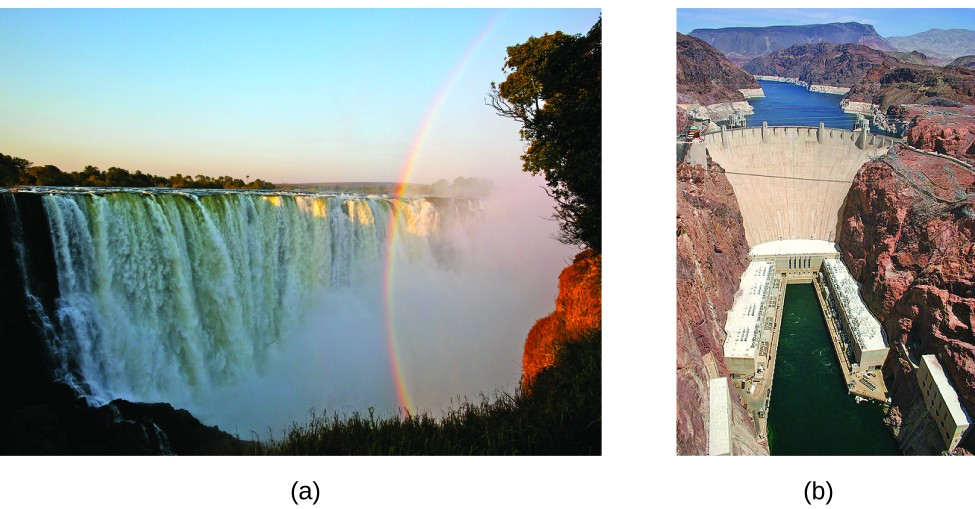Figure 2. (a) Water that is higher in elevation, for example, at the top of Victoria Falls, has a higher potential energy than water at a lower elevation. As the water falls, some of its potential energy is converted into kinetic energy. (b) If the water flows through generators at the bottom of a dam, such as the Hoover Dam shown here, its kinetic energy is converted into electrical energy. (credit a: modification of work by Steve Jurvetson; credit b: modification of work by “curimedia”/Wikimedia commons)

Energy can be converted from one form into another, but all of the energy present before a change occurs always exists in some form after the change is completed. This observation is expressed in the law of conservation of energy: during a chemical or physical change, energy can be neither created nor destroyed, although it can be changed in form. (This is also one version of the first law of thermodynamics, as you will learn later.)

When one substance is converted into another, there is always an associated conversion of one form of energy into another. Heat is usually released or absorbed, but sometimes the conversion involves light, electrical energy, or some other form of energy. For example, chemical energy (a type of potential energy) is stored in the molecules that compose gasoline. When gasoline is combusted within the cylinders of a car’s engine, the rapidly expanding gaseous products of this chemical reaction generate mechanical energy (a type of kinetic energy) when they move the cylinders’ pistons.

According to the law of conservation of matter (seen in an earlier chapter), there is no detectable change in the total amount of matter during a chemical change. When chemical reactions occur, the energy changes are relatively modest and the mass changes are too small to measure, so the laws of conservation of matter and energy hold well. However, in nuclear reactions, the energy changes are much larger (by factors of a million or so), the mass changes are measurable, and matter-energy conversions are significant. This will be examined in more detail in a later chapter on nuclear chemistry. To encompass both chemical and nuclear changes, we combine these laws into one statement: The total quantity of matter and energy in the universe is fixed.

Thermal Energy, Temperature, and Heat

Thermal energy is kinetic energy associated with the random motion of atoms and molecules. Temperature is a quantitative measure of “hot” or “cold.” When the atoms and molecules in an object are moving or vibrating quickly, they have a higher average kinetic energy (KE), and we say that the object is “hot.” When the atoms and molecules are moving slowly, they have lower KE, and we say that the object is “cold” (Figure 3). Assuming that no chemical reaction or phase change (such as melting or vaporizing) occurs, increasing the amount of thermal energy in a sample of matter will cause its temperature to increase. And, assuming that no chemical reaction or phase change (such as condensation or freezing) occurs, decreasing the amount of thermal energy in a sample of matter will cause its temperature to decrease.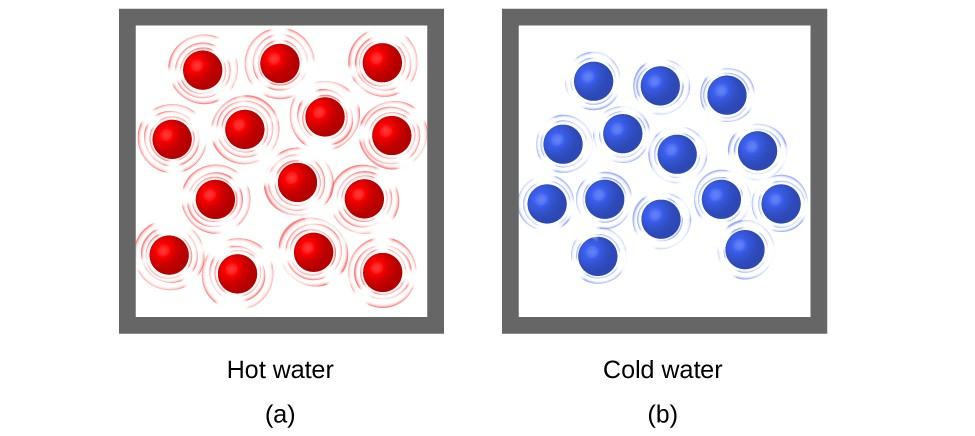Figure 3. (a) The molecules in a sample of hot water move more rapidly than (b) those in a sample of cold water.

Most substances expand as their temperature increases and contract as their temperature decreases. This property can be used to measure temperature changes, as shown in Figure 4. The operation of many thermometers depends on the expansion and contraction of substances in response to temperature changes.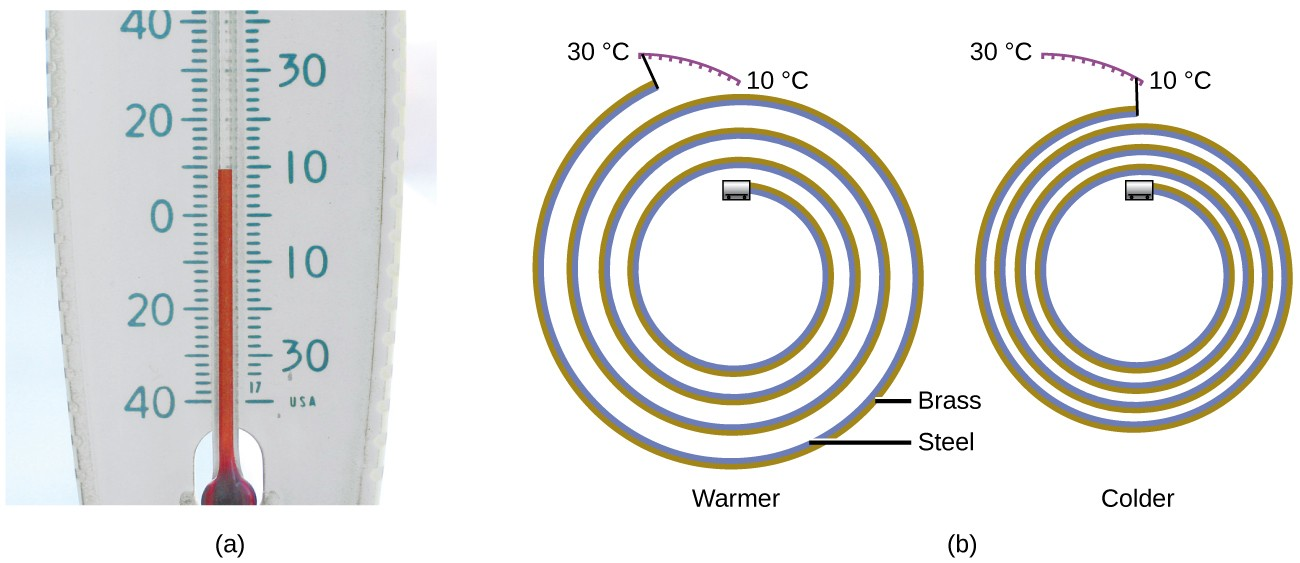Figure 4. (a) In an alcohol or mercury thermometer, the liquid (dyed red for visibility) expands when heated and contracts when cooled, much more so than the glass tube that contains the liquid. (b) In a bimetallic thermometer, two different metals (such as brass and steel) form a two-layered strip. When heated or cooled, one of the metals (brass) expands or contracts more than the other metal (steel), causing the strip to coil or uncoil. Both types of thermometers have a calibrated scale that indicates the temperature. (credit a: modification of work by “dwstucke”/Flickr)

Heat (q) is the transfer of thermal energy between two bodies at different temperatures. Heat flow (a redundant term, but one commonly used) increases the thermal energy of one body and decreases the thermal energy of the other. Suppose we initially have a high temperature (and high thermal energy) substance (H) and a low temperature (and low thermal energy) substance (L). The atoms and molecules in H have a higher average KE than those in L. If we place substance H in contact with substance L, the thermal energy will flow spontaneously from substance H to substance L. The temperature of substance H will decrease, as will the average KE of its molecules; the temperature of substance L will increase, along with the average KE of its molecules. Heat flow will continue until the two substances are at the same temperature (Figure 5).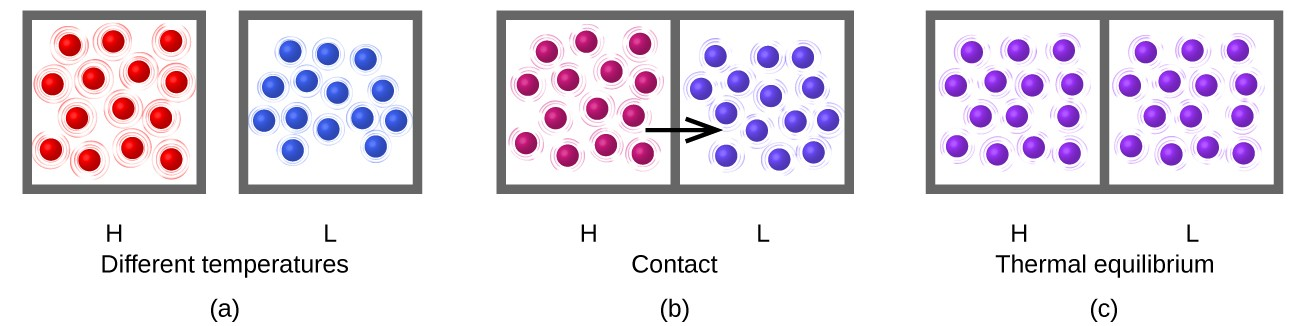Figure 5. (a) Substances H and L are initially at different temperatures, and their atoms have different average kinetic energies. (b) When they are put into contact with each other, collisions between the molecules result in the transfer of kinetic (thermal) energy from the hotter to the cooler matter. (c) The two objects reach “thermal equilibrium” when both substances are at the same temperature, and their molecules have the same average kinetic energy.

Click on the PhET simulation to explore energy forms and changes. Visit the Energy Systems tab to create combinations of energy sources, transformation methods, and outputs. Click on Energy Symbols to visualize the transfer of energy.

Matter undergoing chemical reactions and physical changes can release or absorb heat. A change that releases heat is called an exothermic process. For example, the combustion reaction that occurs when using an oxyacetylene torch is an exothermic process—this process also releases energy in the form of light as evidenced by the torch’s flame (Figure 6). A reaction or change that absorbs heat is an endothermic process. A cold pack used to treat muscle strains provides an example of an endothermic process. When the substances in the cold pack (water and a salt like ammonium nitrate) are brought together, the resulting process absorbs heat, leading to the sensation of cold.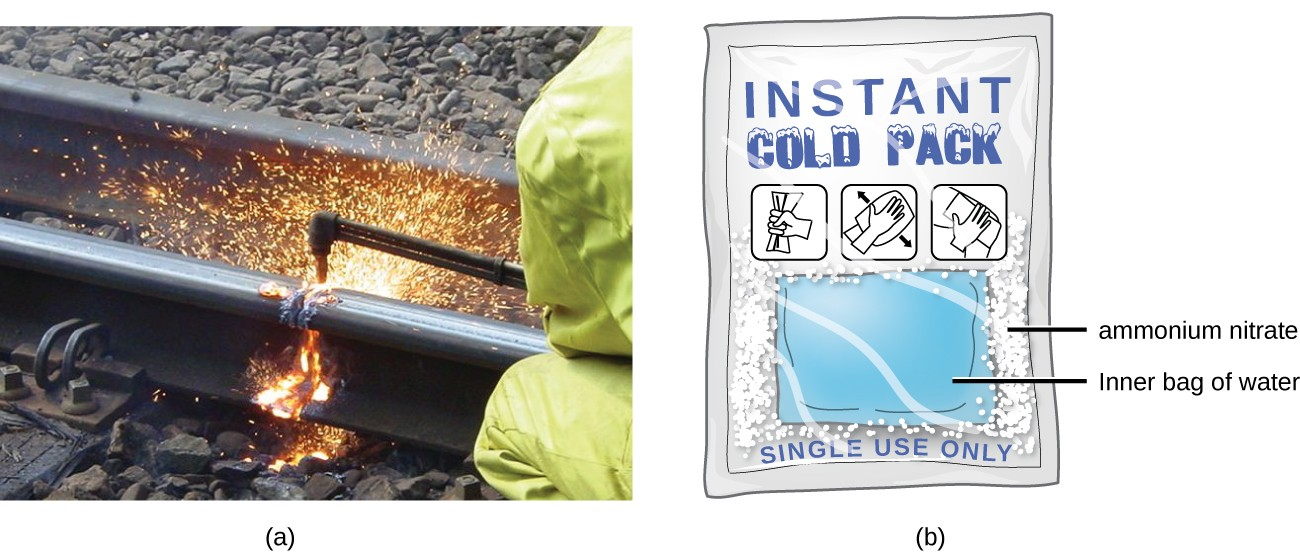Figure 6. (a) An oxyacetylene torch produces heat by the combustion of acetylene in oxygen. The energy released by this exothermic reaction heats and then melts the metal being cut. The sparks are tiny bits of the molten metal flying away. (b) A cold pack uses an endothermic process to create the sensation of cold. (credit a: modification of work by “Skatebiker”/Wikimedia commons)

Historically, energy was measured in units of calories (cal). A calorie is the amount of energy required to raise one gram of water by 1 degree C (1 kelvin). However, this quantity depends on the atmospheric pressure and the starting temperature of the water. The ease of measurement of energy changes in calories has meant that the calorie is still frequently used. The Calorie (with a capital C), or large calorie, commonly used in quantifying food energy content, is a kilocalorie. The SI unit of heat, work, and energy is the joule. A joule (J) is defined as the amount of energy used when a force of 1 newton moves an object 1 meter. It is named in honor of the English physicist James Prescott Joule. One joule is equivalent to 1 kg m2/s2, which is also called 1 newton–meter. A kilojoule (kJ) is 1000 joules. To standardize its definition, 1 calorie has been set to equal 4.184 joules.

We now introduce two concepts useful in describing heat flow and temperature change. The heat capacity (C) of a body of matter is the quantity of heat (q) it absorbs or releases when it experiences a temperature change (ΔT) of 1 degree Celsius (or equivalently, 1 kelvin):

$\displaystyle{C}=\frac{q}{\Delta T}$

Heat capacity is determined by both the type and amount of substance that absorbs or releases heat. It is therefore an extensive property—its value is proportional to the amount of the substance.

For example, consider the heat capacities of two cast iron frying pans. The heat capacity of the large pan is five times greater than that of the small pan, because, although both are made of the same material, the mass of the large pan is five times greater than the mass of the small pan. More mass means more atoms are present in the larger pan, so it takes more energy to make all of those atoms vibrate faster. The heat capacity of the small cast iron frying pan is found by observing that it takes 18,150 J of energy to raise the temperature of the pan by 50.0 °C:

${C}_{\text{small pan}}=\frac{\text{18,140 J}}{50.0^{\circ}\text{ C}}=363\text{ J/}^{\circ}\text{C}$

The larger cast iron frying pan, while made of the same substance, requires 90,700 J of energy to raise its temperature by 50.0 °C. The larger pan has a (proportionally) larger heat capacity, because the larger amount of material requires a (proportionally) larger amount of energy to yield the same temperature change:

${C}_{\text{large pan}}=\frac{\text{90,700 J}}{50.0^{\circ}\text{ C}}=1814\text{ J/}^{\circ}\text{C}$

The specific heat capacity (c) of a substance, commonly called its “specific heat,” is the quantity of heat required to raise the temperature of 1 gram of a substance by 1 degree Celsius (or 1 kelvin):

$\displaystyle{c}=\frac{q}{\text{m}\Delta\text{T}}$

Specific heat capacity depends only on the kind of substance absorbing or releasing heat. It is an intensive property—the type, but not the amount, of the substance is all that matters. For example, the small cast iron frying pan has a mass of 808 g. The specific heat of iron (the material used to make the pan) is therefore:

$\displaystyle{c}_{\text{iron}}=\frac{\text{18,140 J}}{\left(\text{808 g}\right)\left(50.0^{\circ}\text{ C}\right)=\text{0.449 J/g}^{\circ}\text{ C}}$

The large frying pan has a mass of 4040 g. Using the data for this pan, we can also calculate the specific heat of iron:

$\displaystyle{c}_{\text{iron}}=\frac{\text{90,700 J}}{\left(\text{4040 g}\right)\left(50.0^{\circ}\text{ C}\right)=\text{0.449 J/g}^{\circ}\text{ C}}$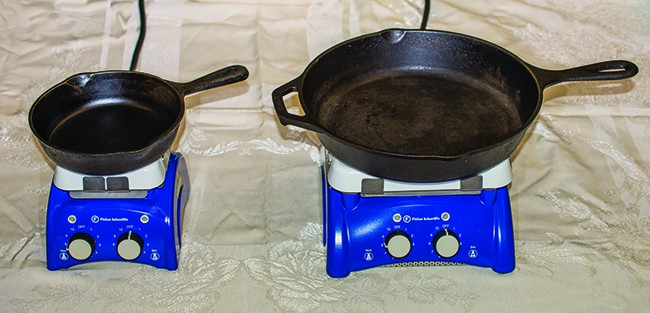Figure 7. Due to its larger mass, a large frying pan has a larger heat capacity than a small frying pan. Because they are made of the same material, both frying pans have the same specific heat. (credit: Mark Blaser)

Although the large pan is more massive than the small pan, since both are made of the same material, they both yield the same value for specific heat (for the material of construction, iron). Note that specific heat is measured in units of energy per temperature per mass and is an intensive property, being derived from a ratio of two extensive properties (heat and mass). The molar heat capacity, also an intensive property, is the heat capacity per mole of a particular substance and has units of J/mol °C (Figure 7).

Liquid water has a relatively high specific heat (about 4.2 J/g °C); most metals have much lower specific heats (usually less than 1 J/g °C). The specific heat of a substance varies somewhat with temperature. However, this variation is usually small enough that we will treat specific heat as constant over the range of temperatures that will be considered in this chapter. Specific heats of some common substances are listed in Table 1.

Table 1. Specific Heats of Common Substances at 25 °C and 1 bar
Substance Symbol (state) Specific Heat (J/g °C)
helium He(g) 5.193
water H2O(l) 4.184
ethanol C2H6O(l) 2.376
ice H2O(s) 2.093 (at -10 °C)
water vapor H2O(g) 1.864
nitrogen N2(g) 1.040
air 1.007
oxygen O2(g) 0.918
aluminum Al(s) 0.897
carbon dioxide CO2(g) 0.853
argon Ar(g) 0.522
iron Fe(s) 0.449
copper Cu(s) 0.385
gold Au(s) 0.129
silicon Si(s) 0.712

If we know the mass of a substance and its specific heat, we can determine the amount of heat, q, entering or leaving the substance by measuring the temperature change before and after the heat is gained or lost:

$\begin{array}{l}q=\left(\text{specific heat}\right)\times \left(\text{mass of substance}\right)\times \left(\text{temperature change}\right)\\ q=c\times m\times \Delta\text{T}=c\times m\times \left({T}_{\text{final}}-{T}_{\text{initial}}\right)\end{array}$

In this equation, c is the specific heat of the substance, m is its mass, and ΔT (which is read “delta T”) is the temperature change, TfinalTinitial. If a substance gains thermal energy, its temperature increases, its final temperature is higher than its initial temperature, TfinalTinitial has a positive value, and the value of q is positive. If a substance loses thermal energy, its temperature decreases, the final temperature is lower than the initial temperature, TfinalTinitial has a negative value, and the value of q is negative.

Example 1: Measuring Heat

A flask containing 8.0 × 102 g of water is heated, and the temperature of the water increases from 21 °C to 85 °C. How much heat did the water absorb?

How much heat, in joules, must be added to a 5.00 × 102-g iron skillet to increase its temperature from 25 °C to 250 °C? The specific heat of iron is 0.451 J/g °C

Note that the relationship between heat, specific heat, mass, and temperature change can be used to determine any of these quantities (not just heat) if the other three are known or can be deduced.

Example 2: Determining Other Quantities

A piece of unknown metal weighs 348 g. When the metal piece absorbs 6.64 kJ of heat, its temperature increases from 22.4 °C to 43.6 °C. Determine the specific heat of this metal (which might provide a clue to its identity).

A piece of unknown metal weighs 217 g. When the metal piece absorbs 1.43 kJ of heat, its temperature increases from 24.5 °C to 39.1 °C. Determine the specific heat of this metal, and predict its identity.

Exercises

Most people find waterbeds uncomfortable unless the water temperature is maintained at about 85 °F. Unless it is heated, a waterbed that contains 892 L of water cools from 85 °F to 72 °F in 24 hours. Estimate the amount of electrical energy required over 24 hours, in kWh, to keep the bed from cooling. Note that 1 kilowatt-hour (kWh) = 3.6 × 106 J, and assume that the density of water is 1.0 g/mL (independent of temperature). What other assumptions did you make? How did they affect your calculated result (i.e., were they likely to yield “positive” or “negative” errors)?

Solar Thermal Energy Power Plants

The sunlight that reaches the earth contains thousands of times more energy than we presently capture. Solar thermal systems provide one possible solution to the problem of converting energy from the sun into energy we can use. Large-scale solar thermal plants have different design specifics, but all concentrate sunlight to heat some substance; the heat “stored” in that substance is then converted into electricity.

The Solana Generating Station in Arizona’s Sonora Desert produces 280 megawatts of electrical power. It uses parabolic mirrors that focus sunlight on pipes filled with a heat transfer fluid (HTF) (Figure 8). The HTF then does two things: It turns water into steam, which spins turbines, which in turn produces electricity, and it melts and heats a mixture of salts, which functions as a thermal energy storage system. After the sun goes down, the molten salt mixture can then release enough of its stored heat to produce steam to run the turbines for 6 hours. Molten salts are used because they possess a number of beneficial properties, including high heat capacities and thermal conductivities.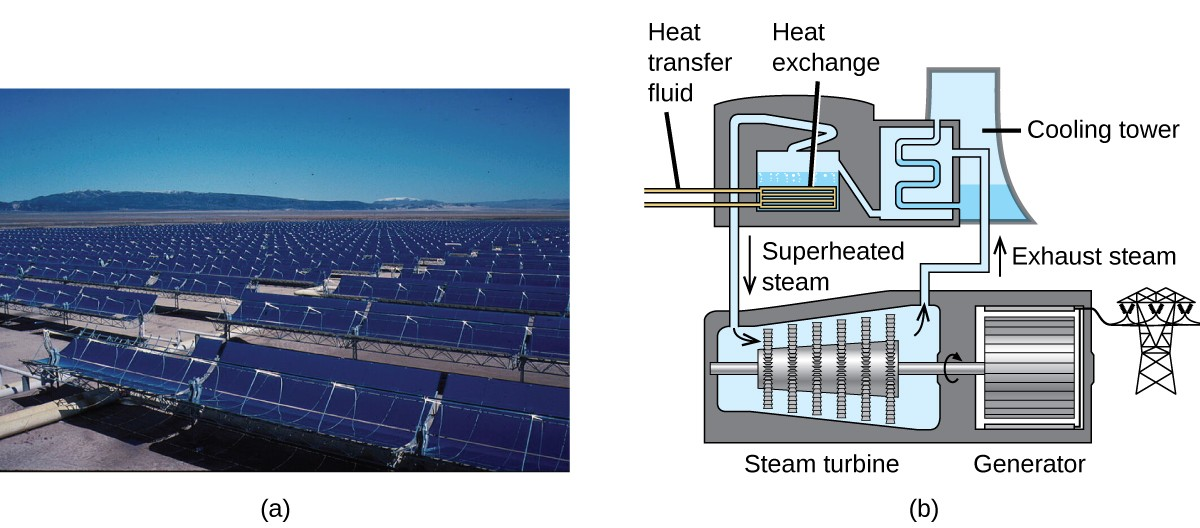Figure 8. This solar thermal plant uses parabolic trough mirrors to concentrate sunlight. (credit a: modification of work by Bureau of Land Management)

The 377-megawatt Ivanpah Solar Generating System, located in the Mojave Desert in California, is the largest solar thermal power plant in the world (Figure 9). Its 170,000 mirrors focus huge amounts of sunlight on three water-filled towers, producing steam at over 538 °C that drives electricity-producing turbines. It produces enough energy to power 140,000 homes. Water is used as the working fluid because of its large heat capacity and heat of vaporization.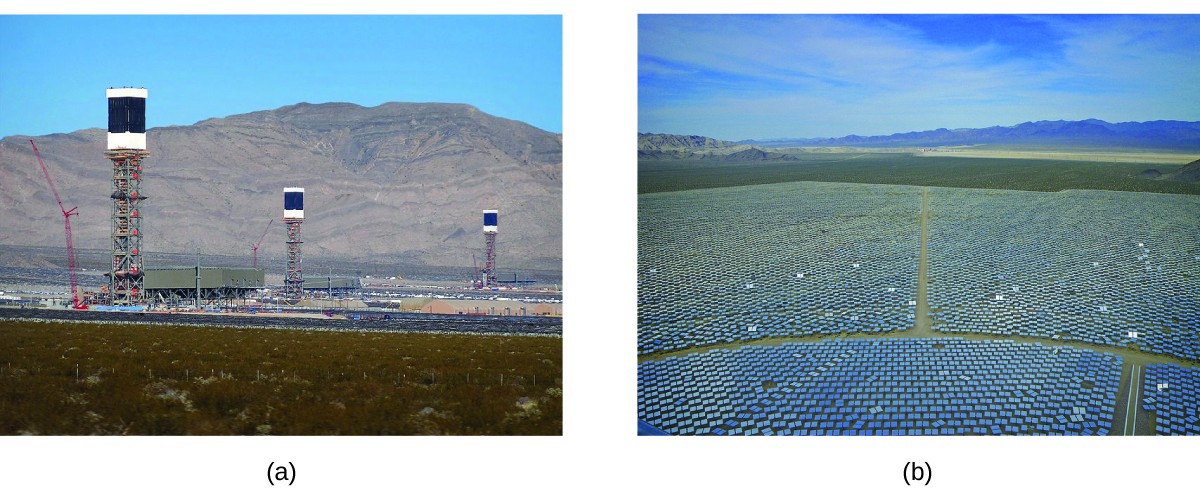Figure 9. (a) The Ivanpah solar thermal plant uses 170,000 mirrors to concentrate sunlight on water-filled towers. (b) It covers 4000 acres of public land near the Mojave Desert and the California-Nevada border. (credit a: modification of work by Craig Dietrich; credit b: modification of work by “USFWS Pacific Southwest Region”/Flickr)

Key Concepts and Summary

Energy is the capacity to do work (applying a force to move matter). Kinetic energy (KE) is the energy of motion; potential energy is energy due to relative position, composition, or condition. When energy is converted from one form into another, energy is neither created nor destroyed (law of conservation of energy or first law of thermodynamics).

Matter has thermal energy due to the KE of its molecules and temperature that corresponds to the average KE of its molecules. Heat is energy that is transferred between objects at different temperatures; it flows from a high to a low temperature. Chemical and physical processes can absorb heat (endothermic) or release heat (exothermic). The SI unit of energy, heat, and work is the joule (J).

Specific heat and heat capacity are measures of the energy needed to change the temperature of a substance or object. The amount of heat absorbed or released by a substance depends directly on the type of substance, its mass, and the temperature change it undergoes.

Key Equations

• $q=c\times m\times \Delta\text{T}=c\times m\times \left({T}_{\text{final}}-{T}_{\text{initial}}\right)$

Exercises

1. A burning match and a bonfire may have the same temperature, yet you would not sit around a burning match on a fall evening to stay warm. Why not?
2. Prepare a table identifying several energy transitions that take place during the typical operation of an automobile.
3. Explain the difference between heat capacity and specific heat of a substance.
4. Calculate the heat capacity, in joules and in calories per degree, of the following:
1. 28.4 g of water
5. Calculate the heat capacity, in joules and in calories per degree, of the following:
1. 45.8 g of nitrogen gas
2. 1.00 pound of aluminum metal
6. How much heat, in joules and in calories, must be added to a 75.0–g iron block with a specific heat of 0.449 J/g °C to increase its temperature from 25 °C to its melting temperature of 1535 °C?
7. How much heat, in joules and in calories, is required to heat a 28.4-g (1-oz) ice cube from -23.0 °C to -1.0 °C?
8. How much would the temperature of 275 g of water increase if 36.5 kJ of heat were added?
9. If 14.5 kJ of heat were added to 485 g of liquid water, how much would its temperature increase?
10. A piece of unknown substance weighs 44.7 g and requires 2110 J to increase its temperature from 23.2 °C to 89.6 °C.
1. What is the specific heat of the substance?
2. If it is one of the substances found in Table 1, what is its likely identity?
11. A piece of unknown solid substance weighs 437.2 g, and requires 8460 J to increase its temperature from 19.3 °C to 68.9 °C.
1. What is the specific heat of the substance?
2. If it is one of the substances found in Table 1, what is its likely identity?
12. An aluminum kettle weighs 1.05 kg.
1. What is the heat capacity of the kettle?
2. How much heat is required to increase the temperature of this kettle from 23.0 °C to 99.0 °C?
3. How much heat is required to heat this kettle from 23.0 °C to 99.0 °C if it contains 1.25 L of water (density of 0.997 g/mL and a specific heat of 4.184 J/g °C)?

Glossary

calorie (cal): unit of heat or other energy; the amount of energy required to raise 1 gram of water by 1 degree Celsius; 1 cal is defined as 4.184 J

endothermic process: chemical reaction or physical change that absorbs heat

energy: capacity to supply heat or do work

exothermic process: chemical reaction or physical change that releases heat

heat (q): transfer of thermal energy between two bodies

heat capacity (C): extensive property of a body of matter that represents the quantity of heat required to increase its temperature by 1 degree Celsius (or 1 kelvin)

joule (J): SI unit of energy; 1 joule is the kinetic energy of an object with a mass of 2 kilograms moving with a velocity of 1 meter per second, 1 J = 1 kg m2/s and 4.184 J = 1 cal

kinetic energy: energy of a moving body, in joules, equal to $\frac{1}{2}m{v}^{2}$ (where m = mass and v = velocity)

potential energy: energy of a particle or system of particles derived from relative position, composition, or condition

specific heat capacity (c): intensive property of a substance that represents the quantity of heat required to raise the temperature of 1 gram of the substance by 1 degree Celsius (or 1 kelvin)

temperature: intensive property of matter that is a quantitative measure of “hotness” and “coldness;” it is related to the average kinetic energy of the atoms or molecules that make up the object

thermal energy: kinetic energy associated with the random motion of atoms and molecules

thermochemistry: study of measuring the amount of heat absorbed or released during a chemical reaction or a physical change

work (w): energy transfer due to changes in external, macroscopic variables such as pressure and volume; or causing matter to move against an opposing force